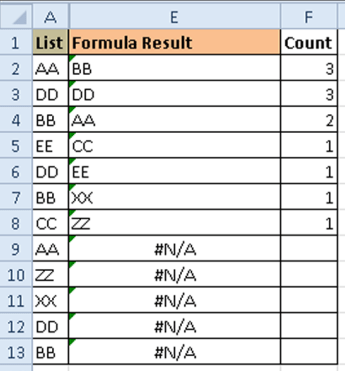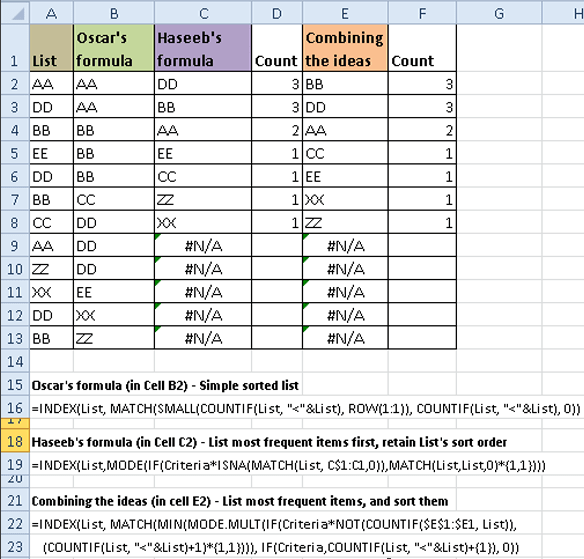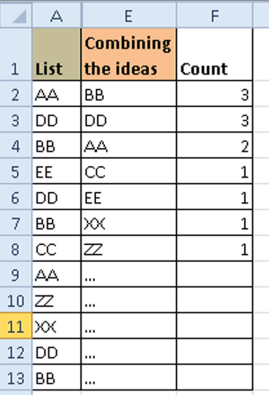# Formula Forensics No. 030 – Extracting a Sorted, Unique List, Grouped by Frequency of Occurrence

This post is the first of hopefully many posts by Guest author Sajan.

Excel offers many ways to sort and group data. (If you have not explored Pivot Tables in Excel, I would highly encourage you to try them out.) However, sometimes it is necessary to be able to control the results using a formula.

The following is a technique to extract a sorted, unique list of items, displaying the most frequently occurring items first, while restricting the output based on some additional criteria.

As always at Formula Forensics you can follow along with a sample file Download Here Excel 2007-13

## The Formula

=INDEX(List, MATCH(MIN(MODE.MULT(IF(Criteria*NOT(COUNTIF(\$E\$1:\$E1, List)), (COUNTIF(List, “<“&List)+1)*{1,1}))), IF(Criteria,COUNTIF(List, “<“&List)+{1}), 0))

Entered into cell E2 with Ctrl+Shift+Enter, and copied down.

(We will add in error checking later.)

Sample results can be seen in following figure:List” is a Named Formula for the source list. (A2:A13 in the example shown.)

Criteria” is a Named Formula for the criteria to apply against the list. For example, (List<> “”)

Disclaimer: Since all of these formulas traverse the source lists, they can get very slow when applied to large lists. I am sharing the formulas more to illustrate the techniques than to endorse them as approaches for every situation. Please determine the suitability for your specific situation.

## Chandoo’s Technique

In an October 2008 article, Chandoo described an ingenious technique of using the COUNTIF() function to sort a list.

http://chandoo.org/wp/2008/10/22/sorting-text-in-excel-using-formulas/

## Oscar’s formula

Oscar Cronquist took it to the next level by describing a formula to create a sorted list using the same technique, in his March, 2009 article:

http://www.get-digital-help.com/2009/03/27/sorting-text-cells-using-array-formula/

=INDEX(List, MATCH(SMALL(COUNTIF(List, “<“&List), ROW(1:1)), COUNTIF(List, “<“&List), 0))

Entered into cell B1 with Ctrl + Shift + Enter, and copied down.

For example, the above formula turns {“DD”; “AA”; “QQ”; “CC”} into {“AA”; “CC”; “DD”; “QQ”}

The heart of Oscar’s formula is the COUNTIF segment where he converts the strings into numbers based on whether a given string is less than other strings in the list. (Please see Oscar’s site for a full explanation of his formula.)

The technique is so simple that you might wonder… why didn’t I think of that?!

That is the sheer genius of the technique!

## Haseeb A’s formula

Recently, Haseeb A provided the following brilliant formula to extract unique items from a list, listing the most frequent items first:

http://chandoo.org/forums/topic/ranking-string-data-for-one-column

=LOOKUP(REPT(“z”,99),CHOOSE({1,2},””,IF(ROWS(E\$4:E4)<=F\$1,INDEX(costcenter,MODE(IF((costcenter<>””)*ISNA(MATCH(costcenter,E\$3:E3,0)),MATCH(costcenter,costcenter,0)*{1,1}))),””)))

Haseeb’s formula returns a value for “Top n” (as specified in cell F\$1).

To make it easy for explanations, I will shorten it by using the same Named Formula “List” as in Oscar’s formula, removing the check for “Top n”, and using the Named Formula “Criteria”:

=INDEX(List,MODE(IF(Criteria*ISNA(MATCH(List, C\$1:C1,0)),MATCH(List,List,0)*{1,1}))) Entered with Ctrl+Shift+Enter into cell C2, and copied down

Haseeb’s formula produces output in the same sequence as the original list, allowing you the flexibility to sort it the way you like it!

For example, the formula turns {“QQ”; “AA”; “XX”; “DD”; “XX”; “DD”; “XX”} into {“XX”; “DD”; “QQ”; “AA”} since “XX” is the most frequently occurring item, followed by “DD”, then “QQ”, then “AA” (the last two presented in the same order as in the source list.)

The formula uses a few different techniques worth calling out:

• ISNA(MATCH(List, C\$1:C1, 0)) is used to skip the items already included in the output. (Please note that the formula is setup in cell C2 and below, while the reference is for the cell up to the previous cell – C1. Also note the use of absolute and relative references to ensure that as the formula gets copied down, the range expands, but still remains anchored on cell C1.)
• MATCH(List, List, 0) is used to convert the strings into numbers (Excel’s forte). The MATCH function returns an array with the location of each string in the list. i.e. if a string is repeated, the same (first) location is returned for both occurrences of the string.
• MATCH(List,List,0)*{1,1} duplicates the result from the MATCH function into column 2 of the array. This is necessary for preventing errors in the MODE function, since MODE does not like it when there are no duplicates in a list. (For example, if List does not have any duplicate strings, MATCH would return a sequential array.)
• The MODE function returns the most frequently occurring number in a list. As such, the MODE(…) segment of the formula returns the most frequently occurring number from MATCH, after skipping the items already displayed in the output. Also, please note that the MATCH function returns the position of a string. As such, the value returned by MODE is the most frequently occurring position in the list.
• Finally, the INDEX function returns the item for the position returned by the MODE function.

A very clever formula! All packed into a small “footprint”!!

## Putting it all Together

Combining the ideas from Chandoo, Oscar, and Haseeb:

Let us now look at my first formula that combines the ideas from Chandoo, Oscar and Haseeb. (i.e. a formula to produce a unique list, sorted alphabetically, and listing the most frequent items first, while restricting the output based on some conditions.)

=INDEX(List, MATCH(MIN(MODE.MULT(IF(Criteria*NOT(COUNTIF(\$E\$1:\$E1, List)), (COUNTIF(List, “<“&List)+1)*{1,1}))), IF(Criteria,COUNTIF(List, “<“&List)+{1}), 0))

Entered into cell E2 with Ctrl+Shift+Enter, and copied down.

In the sample worksheet, Criteria is a named formula set to =(List <> “”)

Later on, we will look at expanding this criterion.

The results from the three formulas can be seen in the following figure.(By the way, the “count” shown in the figure is the count of the adjacent item in the List.)

Let us look at each segment of the formula:

• (COUNTIF(\$E\$1:\$E1, List)) returns an array of numbers where \$E\$1:\$E1 was found in the List. In cell E2, the COUNTIF returns the array “{0;0;0;0;0;0;0;0;0;0;0;0}” indicating that the output(in cell E1:E1, which does not correspond to anything in the List) did not match any values in the List. (In cell E3, COUNTIF(\$E\$1:\$E2, List) returns the array “{0;0;1;0;0;1;0;0;0;0;0;1}” to indicate that matches were found for the string “BB”. Similarly, in cell E4, COUNTIF(\$E\$1:\$E3, List) returns the array “{0;1;1;0;1;1;0;0;0;0;1;1}” to indicate that matches were found for “BB” and “DD”.) Since the output list has each item just once, the COUNTIF function returns zeros or ones. It is also useful to note that the Ones in the returned array correspond to the position of each found item.
• NOT(COUNTIF(\$E\$1:\$E1, List)) reverses the results of the COUNTIF function, switching the zeros and ones. Effectively, the resulting array corresponds to the items from the List that are NOT present in the output.
• Criteria*NOT(COUNTIF(\$E\$1:\$E1, List)) produces an array with zeros and ones, with the ones corresponding to the items in the List that meet the Criteria and are not present in the output. In the sample worksheet, the Criteria is defined as (List<> “” ). One could easily extend the criteria to include additional columns, etc. We will look at an example later in this article.
• COUNTIF(List, “<“&List)+1 returns an array of counts for number of items in the List that are smaller than an item, and increments them by 1. In the sample worksheet, in cell E2, the function returns “{1;7;3;10;7;3;6;1;12;11;7;3}” indicating that 0 items (1-1=0, since we had incremented it) are less than the first item in the list (“AA”), 6 items (7-1=6, since we had incremented it) are smaller than the second item in the list (“DD”), etc. Please note that the function includes duplicates in the counts. The reason for incrementing the results of COUNTIF by 1 is to handle the case where the COUNTIF returns a zero. (The COUNTIF will return a zero when the item is the smallest value in the List.) A zero, while an accurate count, throws the MIN function off, since we do not want MIN to return zero. So, by incrementing all of the values by 1, we keep the accuracy of the order of the results.
• IF(Criteria*NOT(COUNTIF(\$E\$1:\$E1, List)), (COUNTIF(List, “<“&List)+1)*{1,1}) returns an array of counts for the items that are not present in the output, incremented by 1. The multiplication with {1,1} replicates the results of the IF() function into a second column in the array. This duplication is to prevent errors in the MODE function.
• MODE.MULT() returns the most frequently occurring number in a list. If multiple numbers repeat with the same frequency, all of those numbers are returned. For example, for the array {1,2,2,3,2,3,4}, MODE.MULT returns {2} since it is the most frequent item in the array. For the array {1,2,2,3,3,4}, MODE.MULT returns {2,3} since each of them occur with the same frequency. For the array {1,2,3,4}, MODE.MULT returns an error. By multiplying {1;2;3;4} with {1,1}, we get {1,1;2,2;3,3;4,4} creating some duplicates, preventing errors with MODE.MULT.
• MODE.MULT(IF(Criteria*NOT(COUNTIF(\$E\$1:\$E1, List)), (COUNTIF(List, “<“&List)+1)*{1,1})) returns an array of the most frequently occurring counts. For example, in cell E2, the function returns “{7;3}” indicating that 6 and 2 (because we incremented the values) are the most frequently occurring numbers in the array of counts.
• MIN(MODE.MULT(…)) returns the smallest value returned by MODE.MULT. i.e. it returns the number in the earliest position in an alphabetic sort order.
• IF(Criteria,COUNTIF(List, “<“&List)+{1}) returns the counts of items in the list, if the conditions in the Criteria are met. The +{1} forces the result to an array, while incrementing the counts. This is to handle the special case of the List consisting of exactly one item. By adding {1}, we ensure that MATCH() processes its second argument as an array instead of a single value.
• The MATCH(…) function looks up the result of the MIN function ( the lowest value in the sort order) in the count of items in List. The returned value from MATCH provides the location of the matching entry.
• The INDEX(MATCH(…)) returns the value from the location returned by the MATCH function.

Thankfully, the formula is much shorter than the explanation!

### Expanding the Criteria

We can extend the “Criteria” to handle additional conditions. For example, the following figure (Figure 3) indicates column K as showing TRUE or FALSE to indicate whether a certain row in column A should be included in determining the output. (The conditional formatting rule I applied to column A has greyed out those items with a FALSE condition in column K.)I modified the “Criteria” named formula in the sample worksheet to include column K:

=(List<>””)*( \$K\$2:\$K\$13)

### Error Handling

To trap and handle errors, we could wrap the whole formula in an IFERROR().

The formula (in E2), with error handling would become:

=IFERROR(INDEX(List, MATCH(MIN(MODE.MULT(IF(Criteria*NOT(COUNTIF(\$E\$1:\$E1, List)), (COUNTIF(List, “<“&List)+1)*{1,1}))), IF(Criteria,COUNTIF(List, “<“&List)+{1}), 0)), “…”) Ctrl+Shift+Enter

and copied down

Sample results from the worksheet are shown in the following figure:## Final Thoughts

I wish you EXCELlence!

Sajan

## Formula Forensics “The Series”

This is the 30th post in the Formula Forensics series.

Formula Forensic Series

## Formula Forensics Needs Your Help

I need more ideas for future Formula Forensics posts and so I need your help.

If you have a neat formula that you would like to share like above, try putting pen to paper and draft up a Post like Sajan has done above or;

If you have a formula that you would like explained, but don’t want to write a post, send it to Hui or Chandoo.Hello Awesome...

My name is Chandoo. Thanks for dropping by. My mission is to make you awesome in Excel & your work. I live in Wellington, New Zealand. When I am not F9ing my formulas, I cycle, cook or play lego with my kids. Know more about me.

Thank you and see you around.

### Related articles:

 Written by Hui... Tags: array formulas, choose(), countif(), downloads, if(), IIsna(), INDEX(), Learn Excel, lookup, MATCH(), Microsoft Excel Formulas, Mode.mult(), NOT(), row(), small Home: Chandoo.org Main Page ? Doubt: Ask an Excel Question

### 17 Responses to “Formula Forensics No. 030 – Extracting a Sorted, Unique List, Grouped by Frequency of Occurrence”

1.SirJB7 says:

Hi, Sajan!
Great job, congratulations.
Regards!

•Sajan says:

Thanks SirJB7!

Regards,
Sajan.

•Desmond says:

Hello Sajan
I saw this formula in your page however I would like to know the following:
What is "Criteria" here refer to? A list or a number or just a text or anything I can put

=IFERROR(INDEX(List, MATCH(MIN(MODE.MULT(IF(Criteria*NOT(COUNTIF(\$E\$1:\$E1, List)), (COUNTIF(List, “<“&List)+1)*{1,1}))), IF(Criteria,COUNTIF(List, “<“&List)+{1}), 0)), “…”)

•Baljaa says:

Good job,

2.Kyle McGhee says:

Here is what I came up with...it uses a helper column but works just as well.  It does not have anything in the formula regarding criteria conditions.
copy this in Cells C3:C14
=IF(COUNTIF(\$A\$3:A3,A3)>1,"",VALUE(COUNTIF(\$A\$3:\$A\$14,\$A3)&"."&COUNTIF(\$A\$3:\$A\$14,">"&\$A3)))
and copy this in Cells B3:B14
=IFERROR(INDEX(\$A\$3:\$A\$14,MATCH(LARGE(\$C\$3:\$C\$14,ROWS(\$C\$3:C3)),\$C\$3:\$C\$14,0)),"…")
The results are identical to the solution above, posted by Sajan.
The helper column creates a unique list of composite keys of "frequency.Sort order" which is then pulled  into the second formula with the large/index/match functions.

•Sajan says:

Hi Kyle,
Very nice technique!  I recently tried something similar (to generate frequeny.sort order), but used the FREQUENCY() function... needless to say, it made the whole formula unnecessarily complex!

By using the helper column, it would be easy to apply your technique to large data sets also.

One could easily add additional conditions to the helper column formula,  making this technique very extensible.  Nice job!

Thanks for sharing.
Regards,
Sajan.

•Sajan says:

Hi Kyle,
I finally got a chance to try your formula, and I noticed that you would need a slight tweak to your formula for the helper column:
The second COUNTIF() will need to be zero justified so that, for example, 12 would be treated as larger than 9.  i.e., they would need to be formatted to become .12, .09, etc.

You could do that using the TEXT function.
e.g. TEXT(COUNTIF(list, ">" & \$A2), "00")
or more generically,
TEXT(COUNTIF(List,">"&\$A2), REPT("0", LEN(ROWS(List))))

Regards,
Sajan.

•Kyle McGhee says:

Thanks Sajan.  Thanks for pointing that out.  Yes, I forgot about that...I derived the method I posted from another similar excel challenge which also required being zero justified.  This is what I used then:
COUNTIF(\$A\$2:\$A\$13,">"&\$A2)/(10^(LEN(ROWS(\$A\$2:\$A\$13))+1))

Using this changes the formula slightly as the VALUE function is no longer needed.
=IF(COUNTIF(\$A\$3:A3,A3)>1,"",COUNTIF(\$A\$3:\$A\$14,\$A3)+(COUNTIF(\$A\$3:\$A\$14,">"&\$A3)/10^(LEN(ROWS(\$A\$3:\$A\$14)+1))))

3.Ganesh says:

Dear Chandoo.

CR_V00000005
6

CR_V00000005
5

CR_V00000005
4

CR_V00000005
3

CR_V00000005
2

CR_V00000005
1

CR_V00000006
1

CR_V00000007
2

CR_V00000007
1

Like this there are 2000 unique CR numbers with multiple entries ( also 3 more columns with other details belongs to this CR number).  Now i am trying to put serial numbers for these multiple entries. By using Countif function i am getting serial number in reverse order. How can i get these serial number in proper order.

•Ganesh says:

I found the solution

=COUNTIF(A2:A9,A2)-COUNTIF(A3:A9,A2)

thanks

4. [...] may want to read Sajan’s first post here or thank him in the comments [...]

5.anthony says:

Hi,

I have tried to expand the range with a longer list of my own. Unfortunately when I copy down the formula from row 13 onwards the result is the first item on the list all the way down. Can you pls help?

Tks

6.Benzadeus says:

I think that this example is an overkill use of formulas. Why don't just use a pivot table for this case?

•Hui... says:

Formula Forensics does not tell people that this is the only way to solve a problem, and as you have pointed out often there are several ways to solve problems in Excel.

Formula Forensics is about teaching people to solve problems using common and simple Excel functions which are often put together in initially strange and mysterious ways but which can return truly amazing results when understood. I think it is the technique of analysing the problems that Formula Forensics tries to impart on the reader, not the actual solution.

My preference is to always use Formulas for solutions as opposed to Excel functions, purely from the point of view that a Formula based solution will always be up to date as the data changes as opposed to a Pivot table which must be refreshed as the data changes

Hui...

7.cmore says:

I love this explanation, robust. Question I have for you.

Assume I cannot copy and place in other cells and am trying to avoid advanced filter because of the label headings for criteria requirement

Lastly assume that the range/array is currently housed in a named range as an array operation

1. How do I sort Range that contains mixed data in a given cell
so
A
B
1
C
D

2. VBA: What are the options for Selecting and Printing back to workbook items from an VBA array given criteria (unique and distinct) (Copy formula Paste Values?, Evaluate Formula Paste Values, Other?)

So basically, can I do the same thing in VBA because array formula on 15K plus rows is killing the tool's performance and is ultimately affecting how I create the dashboard of resulting data

1) Load Dynamic Range to Array
2.) Create new array from that based on Criteria
3.) Place new array back into workbook?

8.Sam says:

Considering that different data will have different ranges, how would I make your solution more robust so that the 'List' column length doesn't need to be predefined for every new list. Changing "List" from \$A\$2:\$A\$13 to \$A\$2:\$A or even to a higher number, such as \$A\$2:\$A\$999, doesn't work. Thanks, Sam

 « Using pivot tables to find out non performing customers Excel Formatting Tips – Gangnam Style [open thread] »

### Get FREE Excel & Power-BI Newsletter

One email per week with Excel and Power BI goodness. Join 100,000+ others and get it free.